# How to get an int value from Indicator? - page 217411

vx0532:

sorry, I don't know your question exactly, could you told me how shall I modify my codes?

if I don't assign it a value, I think Call is 0.0? yes?

No, it's EMPTY_VALUE . . . which is not 0.0 so your test . . .

`if(Get_High != 0 && OrdersTotal() == 0)`

is not the same as the one in the EA without the iCustom() call . . .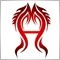1141

RaptorUK:

No, it's EMPTY_VALUE . . . which is not 0.0 so your test . . .

is not the same as the one in the EA without the iCustom() call . . .

Thank you

Now I want to modify the EA which call iCustom() to be the same as the EA which doesn't call iCustom().

could you modify my code?17411

vx0532:

Thank you

Now I want to modify the EA which call iCustom() to be the same as the EA which doesn't call iCustom().

could you modify my code?

Yes I could, but you cannot afford me . . . you do it and you will learn while doing it.25849

learn to code it, or pay someone. We're not going to code it FOR you. We are willing to HELP you when you post your attempt (using SRC) and the nature of your problem.1141

RaptorUK:

No, it's EMPTY_VALUE . . . which is not 0.0 so your test . . .

is not the same as the one in the EA without the iCustom() call . . .

Thank you

I have test it, yes, it's Value is 0;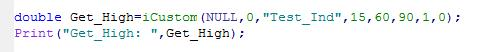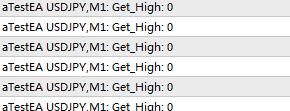if(Get_High != 0 && OrdersTotal() == 0) this code is the exactly the same in EA(without calling indicator) and EA(calling indicator),please confirm this1141

I have modified these codes a little to find some thing important.

the EA without Indicator's code is as below:

```extern int Seek_Period=15,Mini_Swing=60,Trade_Range=90;
int Stop_Loss=80;
double Get_High;

int init()
{
return(0);
}
int deinit()
{
return(0);
}

int start()
{
Get_High_Low();
if(MathAbs(Get_High-1.0)<Point)
Print("Get_High:",Get_High);
if(MathAbs(Get_High-1.0)<Point&&OrdersTotal()==0)
OrderSend(Symbol(),OP_SELL,1,Bid,3,Bid+Stop_Loss*Point,Bid-3*Stop_Loss*Point,0,0,0,0);
return(0);
}

void Get_High_Low()
{
int P_CNT=Seek_Period;
Get_High=0.0;
int G_i=0;
while(P_CNT>=6)
{
G_i=iHighest(NULL,0,MODE_HIGH,P_CNT,0);
double High_Refer_P=High[iHighest(NULL,0,MODE_HIGH,10,G_i-5)],Low_Refer_P=Low[iLowest(NULL,0,MODE_LOW,G_i,0)];
double HL_Current_P=High[iHighest(NULL,0,MODE_HIGH,3,0)];
if(
G_i>=5&&MathAbs(High_Refer_P-High[G_i])<Point&&High[G_i]-Low_Refer_P>=Mini_Swing*Point&&HL_Current_P!=High
)  {Get_High=1.0;break;}

P_CNT--;
}
return;
}
```

the EA with calling Indicator 's code is as below:

```int Stop_Loss=80;
double Get_High;

int init()
{
return(0);
}

int deinit()
{
return(0);
}

int start()
{
if(MathAbs(Get_High-1.0)<Point)
Print("Get_High:",Get_High);
Get_High=iCustom(NULL,0,"Test_Ind",15,60,90,1,0);
if(MathAbs(Get_High-1.0)<Point&&OrdersTotal()==0)
OrderSend(Symbol(),OP_SELL,1,Bid,3,Bid+Stop_Loss*Point,Bid-3*Stop_Loss*Point,0,0,0,0);
return(0);
}```

Indicator(Test_Ind):

```#property indicator_chart_window
#property indicator_buffers 1
#property indicator_color1 Yellow

double High_Point[];
double Call;

int init()
{
IndicatorBuffers(2);
SetIndexBuffer(0,High_Point);
SetIndexStyle(0,DRAW_SECTION);
SetIndexEmptyValue(0,0.0);
SetIndexBuffer(1,Call);
return(0);
}
int deinit()
{
return(0);
}

int start()
{
if(Bars<=Seek_Period) return(0);
int Counted_Bars=IndicatorCounted();
if(Counted_Bars==0) Counted_Bars--;
int Limit=Bars-Counted_Bars;
ArrayInitialize(Call,0.0);
for(int i=Limit-1;i>0;i--)
{
int P_CNT=Seek_Period;
int G_i=0;
while(P_CNT>=6)
{
G_i=iHighest(NULL,0,MODE_HIGH,P_CNT,0);
double High_Refer_P=High[iHighest(NULL,0,MODE_HIGH,10,G_i-5)],Low_Refer_P=Low[iLowest(NULL,0,MODE_LOW,G_i,0)];
double HL_Current_P=High[iHighest(NULL,0,MODE_HIGH,3,0)];
if(
G_i>=5&&MathAbs(High_Refer_P-High[G_i])<Point&&High[G_i]-Low_Refer_P>=Mini_Swing*Point&&HL_Current_P!=High
)  {Call=1.0;break;}
P_CNT--;
}
}

return(0);
}
```
`the EA without calling Indicator's Journal is as below: why there is not the notes which show information of open order, close order and so on`
```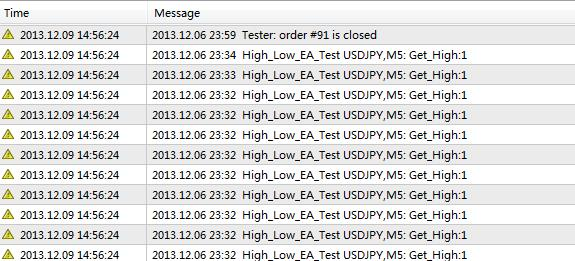```
`The EA with calling Indicator' journal is as below: I think the type journal is Ok, but orders are so few when compared to the EA without calling Indicator's journal`
```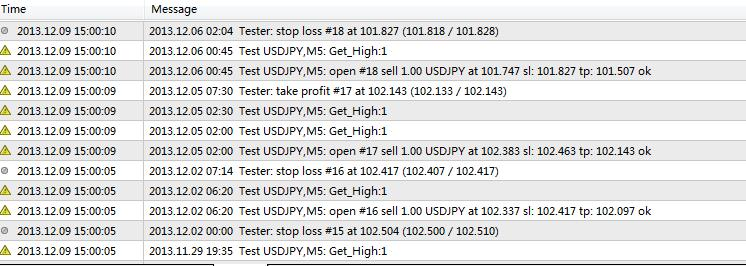```